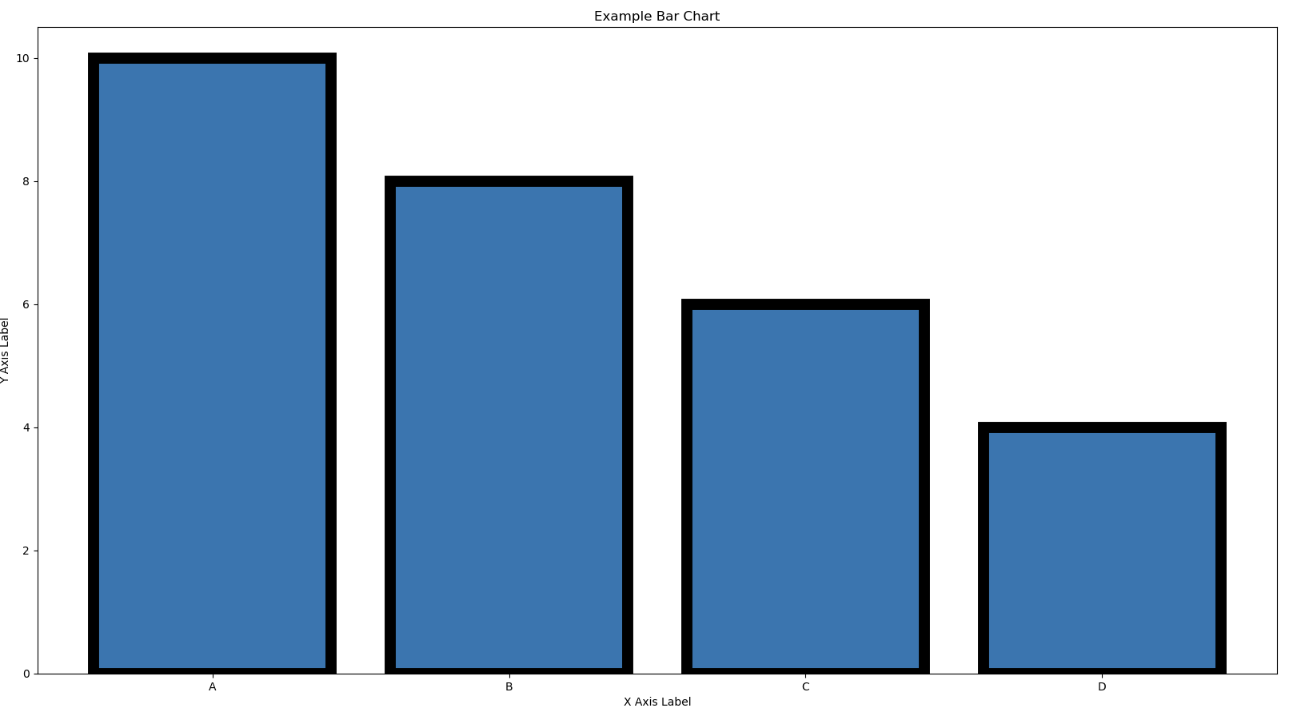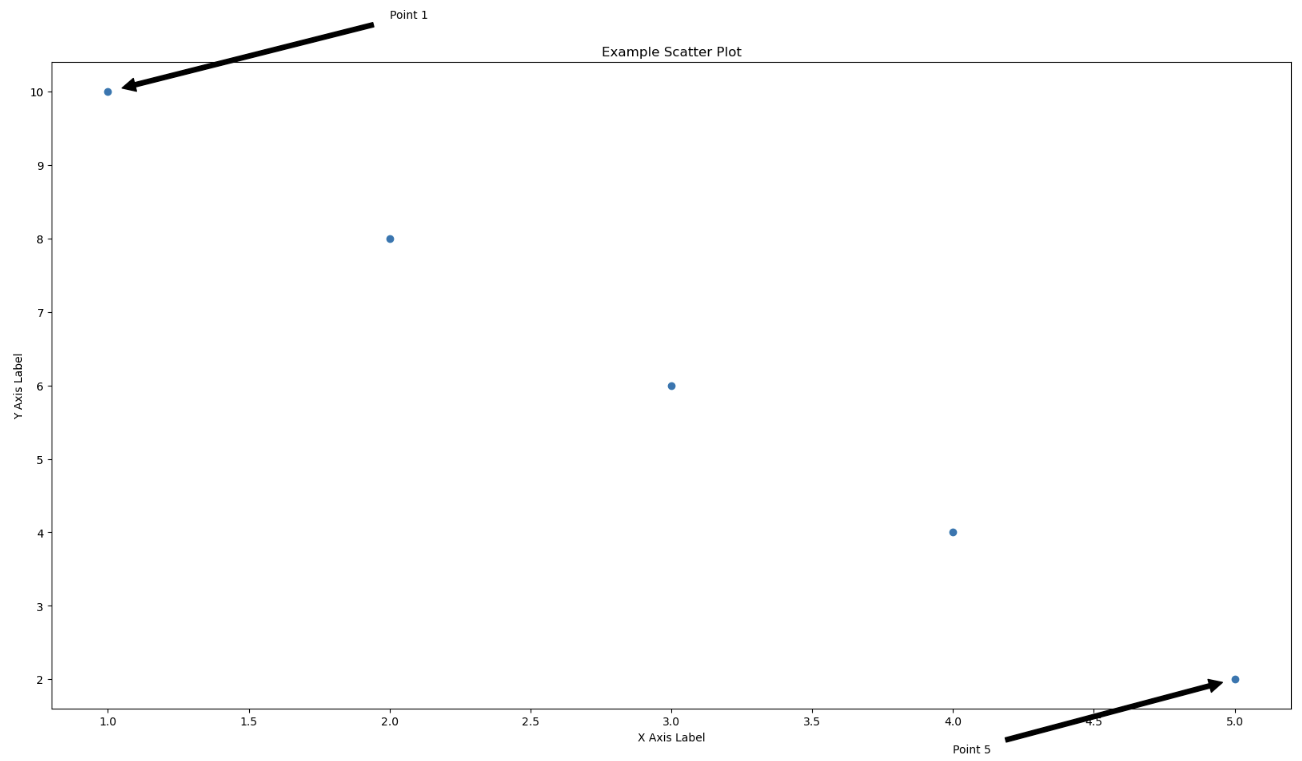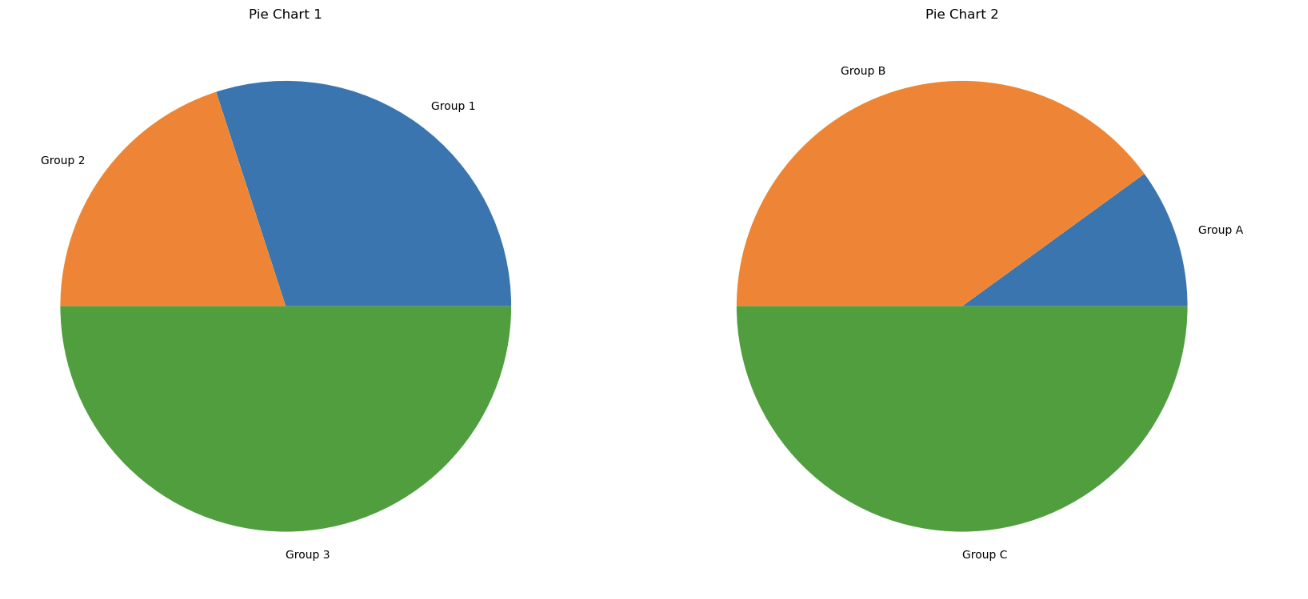# Three ways to make your Python Viz stand out and impress!

Working with data means you are visualising, visualising, visualising

Wether that means visualising exploratory analysis, results, insights, reports, the Gucci Flip Flops you're going to buy once you get your first tech job, visualisations are hugely important.Adding edges to a bar chart can really add emphasis to your viz and help it standout. It is also a super simple method of making a viz seem far different to the default without really doing too much.

In this example, we use the 'edgecolor' parameter to specify the color of the borders around each bar. By setting it to 'black', we make the borders black. You can also adjust the width of the borders using the 'linewidth' parameter.

```import matplotlib.pyplot as plt

# Sample data
x = ['A', 'B', 'C', 'D']
y = [10, 8, 6, 4]

# Create a bar chart with black borders
plt.bar(x, y, edgecolor='black', linewidth = 10)

# Set the chart title and axis labels
plt.title('Example Bar Chart')
plt.xlabel('X Axis Label')
plt.ylabel('Y Axis Label')

# Show the chart
plt.show()
```# 2. Adding annotations to a scatterplot:

Including annotations on a scatterplot or any graph is a fantastic way to draw attention quickly to a certain point in your data. This is great when you want to showcase a marked improvement in time or performance. Also a great way to pinpoint a change in external or internal factors that you're trying to get across. You want your visualisations to be direct, and your audience able to understand the information quickly.

```
import matplotlib.pyplot as plt

# Sample data
x = [1, 2, 3, 4, 5]
y = [10, 8, 6, 4, 2]

# Create a scatter plot
plt.scatter(x, y)

# Add text annotations to the plot
plt.annotate('Point 1', xy=(1, 10), xytext=(2, 11),
arrowprops=dict(facecolor='black', shrink=0.05))
plt.annotate('Point 5', xy=(5, 2), xytext=(4, 1),
arrowprops=dict(facecolor='black', shrink=0.05))

# Set the chart title and axis labels
plt.title('Example Scatter Plot')
plt.xlabel('X Axis Label')
plt.ylabel('Y Axis Label')

# Show the plot
plt.show()

```# 3. Double pie charts :

Having multiple bar charts or scatterplots together can be jarring and confusing for your audience but two pie charts together can be a lot easier on the eye.

In this example, we use the subplots function to create a figure with two subplots, and then we assign them to ax1 and ax2. We then create the two pie charts on their respective subplots using the pie function. Finally, we add titles to each of the charts using the set_title method. You can make a lot more simple alterations such as adjusting the sizes and labels of the pie charts or adding more subplots.

```
import matplotlib.pyplot as plt

# Sample data for the first pie chart
sizes1 = [30, 20, 50]
labels1 = ['Group 1', 'Group 2', 'Group 3']

# Sample data for the second pie chart
sizes2 = [10, 40, 50]
labels2 = ['Group A', 'Group B', 'Group C']

# Create a figure with two subplots
fig, (ax1, ax2) = plt.subplots(1, 2)

# Create the first pie chart
ax1.pie(sizes1, labels=labels1)

# Add a title to the first pie chart
ax1.set_title('Pie Chart 1')

# Create the second pie chart
ax2.pie(sizes2, labels=labels2)

# Add a title to the second pie chart
ax2.set_title('Pie Chart 2')

# Display the charts side by side
plt.show()

```# So What have we learned ?

The biggest take home for me is that you can do a lot with very little in this space. A small addition like an annotation or some more emphasis on the edges can do a lot to draw attention and make your visualisations memorable.